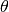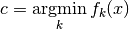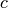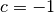# secml.ml.classifiers.reject¶

## CClassifierReject¶

class secml.ml.classifiers.reject.c_classifier_reject.CClassifierReject(preprocess=None)[source]

Abstract class that defines basic methods for Classifiers with reject.

A classifier assign a label (class) to new patterns using the informations learned from training set.

This interface implements a set of generic methods for training and classification that can be used for every algorithms. However, all of them can be reimplemented if specific routines are needed.

Parameters
preprocessstr or CNormalizer

Features preprocess to applied to input data. Can be a CNormalizer subclass or a string with the desired preprocess type. If None, input data is used as is.

Attributes
class_type

Defines class type.

classes

Return the list of classes on which training has been performed.

logger

Logger for current object.

n_classes

Number of classes of training dataset.

n_features

Number of features (before preprocessing).

preprocess

Inner preprocessor (if any).

verbose

Verbosity level of logger output.

Methods

 backward(self[, w]) Returns the preprocessor gradient wrt data. copy(self) Returns a shallow copy of current class. create([class_item]) This method creates an instance of a class with given type. decision_function(self, x[, y]) Computes the decision function for each pattern in x. deepcopy(self) Returns a deep copy of current class. estimate_parameters(self, dataset, …[, …]) Estimate parameter that give better result respect a chose metric. fit(self, dataset[, n_jobs]) Trains the classifier. forward(self, x[, caching]) Forward pass on input x. get_class_from_type(class_type) Return the class associated with input type. get_params(self) Returns the dictionary of class parameters. get_state(self) Returns the object state dictionary. get_subclasses() Get all the subclasses of the calling class. grad_f_x(self, x, y) Computes the gradient of the classifier’s decision function wrt x. gradient(self, x[, w]) Compute gradient at x by doing a backward pass. is_fitted(self) Return True if the classifier is trained (fitted). list_class_types() This method lists all types of available subclasses of calling one. load(path) Loads object from file. load_state(self, path) Sets the object state from file. predict(self, x[, return_decision_function, …]) Perform classification of each pattern in x. save(self, path) Save class object to file. save_state(self, path) Store the object state to file. set(self, param_name, param_value[, copy]) Set a parameter of the class. set_params(self, params_dict[, copy]) Set all parameters passed as a dictionary {key: value}. set_state(self, state_dict[, copy]) Sets the object state using input dictionary. timed([msg]) Timer decorator.
abstract predict(self, x, return_decision_function=False, n_jobs=1)[source]

Perform classification of each pattern in x.

If a preprocess has been specified, input is normalized before classification.

Parameters
xCArray

Array with new patterns to classify, 2-Dimensional of shape (n_patterns, n_features).

return_decision_functionbool, optional

Whether to return the decision_function value along with predictions. Default False.

n_jobsint, optional

Number of parallel workers to use for classification. Default 1. Cannot be higher than processor’s number of cores.

Returns
labelsCArray

Flat dense array of shape (n_patterns,) with the label assigned to each test pattern. The classification label is the label of the class associated with the highest score. The rejected samples have label -1.

scoresCArray, optional

Array of shape (n_patterns, n_classes) with classification score of each test pattern with respect to each training class. Will be returned only if return_decision_function is True.

## CClassifierRejectThreshold¶

class secml.ml.classifiers.reject.c_classifier_reject_threshold.CClassifierRejectThreshold(clf, threshold, preprocess=None)[source]
Abstract class that defines basic methods for Classifiers with reject

based on a certain threshold.

A classifier assign a label (class) to new patterns using the informations learned from training set.

The samples for which the higher score is under a certain threshold are rejected by the classifier.

Parameters
clfCClassifier

Classifier to which we would like to apply a reject threshold. The classifier can also be already fitted. In this case, if a preprocessor was used during fitting, the same preprocessor must be passed to the outer classifier.

thresholdfloat

Rejection threshold.

preprocessCPreProcess or str or None, optional

Features preprocess to be applied to input data. Can be a CPreProcess subclass or a string with the type of the desired preprocessor. If None, input data is used as is.

Attributes
class_type

Defines class type.

classes

Return the list of classes on which training has been performed.

clf

Returns the inner classifier.

logger

Logger for current object.

n_classes

Number of classes of training dataset, plus the rejection class.

n_features

Number of features (before preprocessing).

preprocess

Inner preprocessor (if any).

threshold

Returns the rejection threshold.

verbose

Verbosity level of logger output.

Methods

 backward(self[, w]) Returns the preprocessor gradient wrt data. copy(self) Returns a shallow copy of current class. create([class_item]) This method creates an instance of a class with given type. decision_function(self, x[, y]) Computes the decision function for each pattern in x. deepcopy(self) Returns a deep copy of current class. estimate_parameters(self, dataset, …[, …]) Estimate parameter that give better result respect a chose metric. fit(self, dataset[, n_jobs]) Trains the classifier. forward(self, x[, caching]) Forward pass on input x. get_class_from_type(class_type) Return the class associated with input type. get_params(self) Returns the dictionary of class parameters. get_state(self) Returns the object state dictionary. get_subclasses() Get all the subclasses of the calling class. grad_f_x(self, x, y) Computes the gradient of the classifier’s decision function wrt x. gradient(self, x[, w]) Compute gradient at x by doing a backward pass. is_fitted(self) Return True if the classifier is trained (fitted). list_class_types() This method lists all types of available subclasses of calling one. load(path) Loads object from file. load_state(self, path) Sets the object state from file. predict(self, x[, return_decision_function, …]) Perform classification of each pattern in x. save(self, path) Save class object to file. save_state(self, path) Store the object state to file. set(self, param_name, param_value[, copy]) Set a parameter of the class. set_params(self, params_dict[, copy]) Set all parameters passed as a dictionary {key: value}. set_state(self, state_dict[, copy]) Sets the object state using input dictionary. timed([msg]) Timer decorator.
property classes

Return the list of classes on which training has been performed.

property clf

Returns the inner classifier.

fit(self, dataset, n_jobs=1)[source]

Trains the classifier.

If a preprocess has been specified, input is normalized before training.

Parameters
datasetCDataset

Training set. Must be a CDataset instance with patterns data and corresponding labels.

n_jobsint, optional

Number of parallel workers to use for training the classifier. Default 1. Cannot be higher than processor’s number of cores.

Returns
trained_clsCClassifier

Instance of the classifier trained using input dataset.

property n_classes

Number of classes of training dataset, plus the rejection class.

predict(self, x, return_decision_function=False, n_jobs=<no value>)[source]

Perform classification of each pattern in x.

The score matrix of this classifier is equal to the predicted outputs plus a column (corresponding to the reject class) with all its values equal to, beingthe reject threshold.

The predicted class is therefore:wherecorrespond to the rejection class (i.e.,) only when the maximum taken over the other classes (excluding the reject one) is not greater than the reject threshold.

If a preprocess has been specified, input is normalized before classification.

Parameters
xCArray

Array with new patterns to classify, 2-Dimensional of shape (n_patterns, n_features).

return_decision_functionbool, optional

Whether to return the decision_function value along with predictions. Default False.

n_jobsint, optional

Number of parallel workers to use for classification. Default _NoValue. Cannot be higher than processor’s number of cores.

Returns
labelsCArray

Flat dense array of shape (n_patterns,) with the label assigned to each test pattern. The classification label is the label of the class associated with the highest score. The samples for which the label is equal -1 are the ones rejected by the classifier

scoresCArray, optional

Array of shape (n_patterns, n_classes) with classification score of each test pattern with respect to each training class. Will be returned only if return_decision_function is True.

property threshold

Returns the rejection threshold.